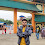# HackerRank What's your name solution in Python

In this HackerRank What's your name problem solution in python, You are given the firstname and lastname of a person on two different lines. Your task is to read them and print the following:

Hello firstname lastname! You just delved into python.

>

## Problem solution in Python 2 programming.

```def full_name(f,s):
return 'Hello %s %s! You just delved into python.' % (f,s)

first = raw_input()
second = raw_input()

print full_name(first, second)```

## Problem solution in Python 3 programming.

```def print_full_name(a, b):
print("Hello {} {}! You just delved into python.".format(a,b))```

### Problem solution in pypy programming.

```# Enter your code here. Read input from STDIN. Print output to STDOUT
fn = raw_input()
ln = raw_input()
print("Hello {} {}! You just delved into python.".format(fn, ln))```

### Problem solution in pypy3 programming.

```# Enter your code here. Read input from STDIN. Print output to STDOUT
first_name = input()
last_name = input()
output = "Hello {0} {1}! You just delved into python.".format(first_name,last_name)
print(output)```

1.1.2.